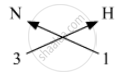SSC (English Medium) Class 8Maharashtra State Board
Share

# Deduce the Molecular Formulae of the Compound Obtained from the Following Pairs of Elements by the Cross Multiplication Method. - SSC (English Medium) Class 8 - Science

ConceptMolecular Formula and Valency

#### Question

Deduce the molecular formulae of the compound obtained from the following pairs of elements by the cross multiplication method.

N (Valency 3) & H (Valency 1)

#### Solution

N (Valency 3) & H (Valency 1)
Step 1 : Write the symbols of the radicals.
N                      H
Step 2 : Write the valency below the respective radical.
N                      H
3                      1
Step 3 : Cross-multiply symbols of radicals with their respective valency.Step 4 : Write down the chemical formula of the compound.

NH3

Is there an error in this question or solution?

#### APPEARS IN

Balbharati Solution for Balbharati Class 8 Science - General Science (2018 to Current)
Chapter 6: Composition of Matter
Exercise | Q: 8.2 | Page no. 47
Solution Deduce the Molecular Formulae of the Compound Obtained from the Following Pairs of Elements by the Cross Multiplication Method. Concept: Molecular Formula and Valency.
S